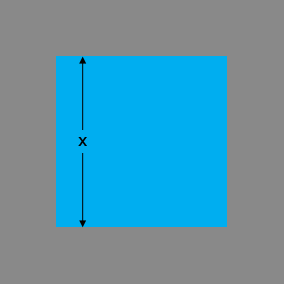SEARCH HOMEMath Central Quandaries & QueriesQuestion from Maddie, a student: A square Water Fountain is Surrounded by a walk 2 yards wide. If the Area of the Fountain is 2/3 of the total area of the Fountain and the Walk. What are the Outside Dimensions of the WalkDraw a diagram!Suppose the fountain is $x$ yards by $x$ yards. What is its area?
What are the dimensions of the fountain plus the walk? What is the area of the fountain plus the walk?
The area of the fountain is $\frac23$ this area.

Solve for $x.$

PennyMath Central is supported by the University of Regina and The Pacific Institute for the Mathematical Sciences.